1350字，阅读需时5分钟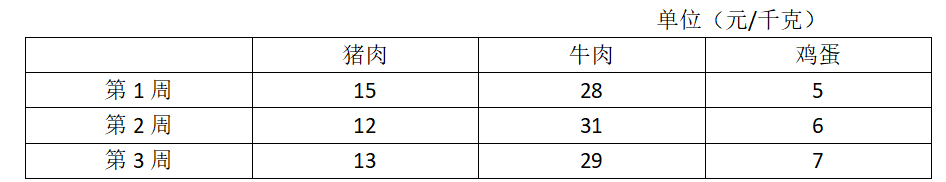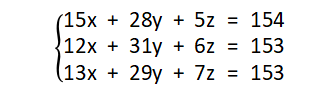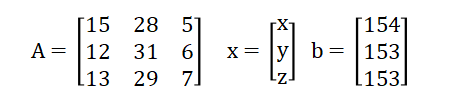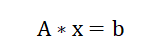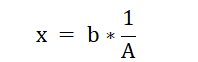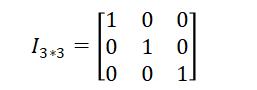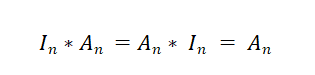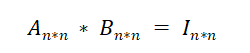A的逆矩阵被记为：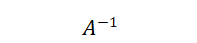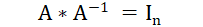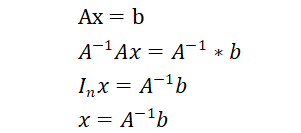# 导入numpy库
import numpy as np

# 定义矩阵A
A = np.array([[15,28,5],
[12,31,6],
[13,29,7]
])
# 定义矩阵b
b = np.array([[154,153,153]])

# 计算矩阵A的逆矩阵inverseA
inverseA = np.linalg.inv(A)
# 输出A与其逆矩阵的乘积
print(np.matmul(inverseA,A))

# 求解x
x = np.matmul(inverseA,np.transpose(b))
# 输出解x
print(x)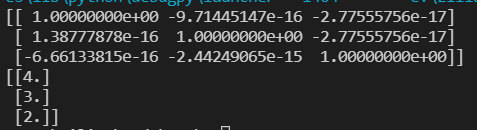n阶矩阵的逆矩阵也称为非奇异矩阵，否则称为奇异矩阵。判断一个n阶矩阵A是否可逆，要看矩阵A的行列式|A|是否等于零，若等于零，则矩阵A为奇异矩阵，否则为非奇异矩阵。若A为奇异矩阵，则AX=0有无穷解，AX=b有无穷解或者无解。若A为非奇异矩阵，则AX=0有且只有唯一零解，AX=b有唯一解